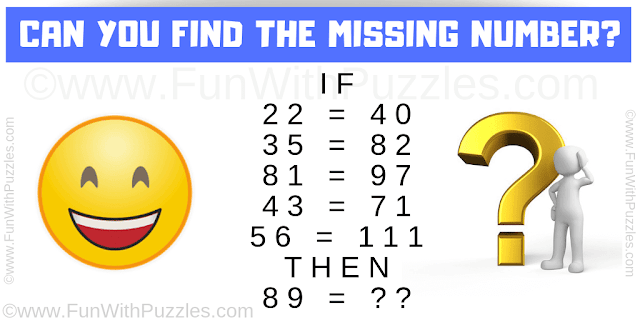This is very interesting maths logical reasoning question which will make you scratch your head. In this maths logic puzzle, there are some number equations which are mathematically incorrect. However, these number equations follow a certain logical pattern. Your challenge is to decode the logical reasoning hidden in these equations and then solve the last equation to find the missing number.Can you solve this Maths Logical Reasoning Question?

Answer of this "Maths Logical Reasoning Question", can be viewed by clicking on the answer button.

Ash said...

IF.

43+71 =21

52+73=30

49+81-81

32+89=??

Then,

Rajesh Kumar said...

@Ash,
I could not solve this one. It will be great if you can post the logical reasoning used in this puzzle?